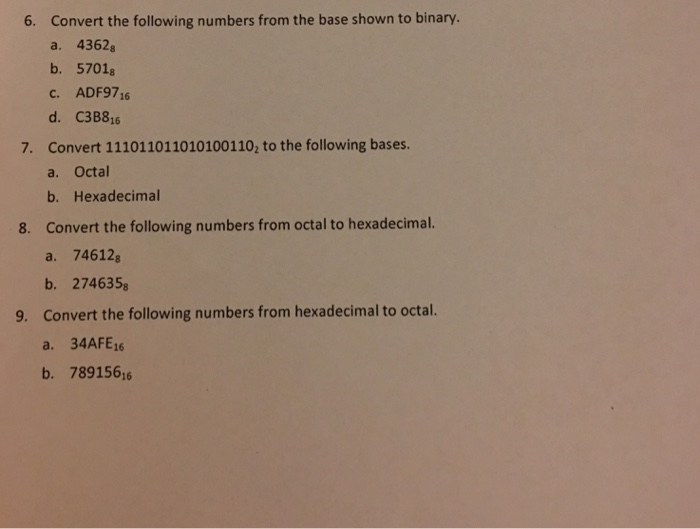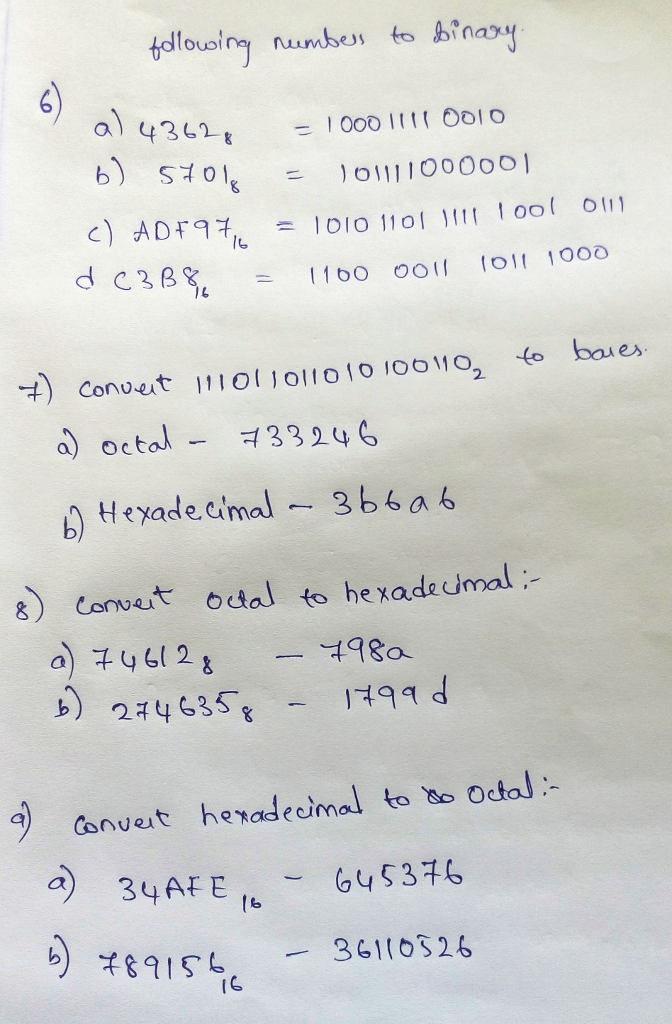# Question & Answer: Convert the following numbers from the base shown to binary. a. 4362_8 b. 5701_8 c. ADF97_16 d. C3B8_16 7. Con…..Convert the following numbers from the base shown to binary. a. 4362_8 b. 5701_8 c. ADF97_16 d. C3B8_16 7. Convert 111011011010100110_2 to the following bases. a. Octal b. Hexadecimal Convert the following numbers from octal to hexadecimal. a. 74612_8 b. 274635_8 Convert the following numbers from hexadecimal to octal. a. 34AFE_16 b. 789156_16